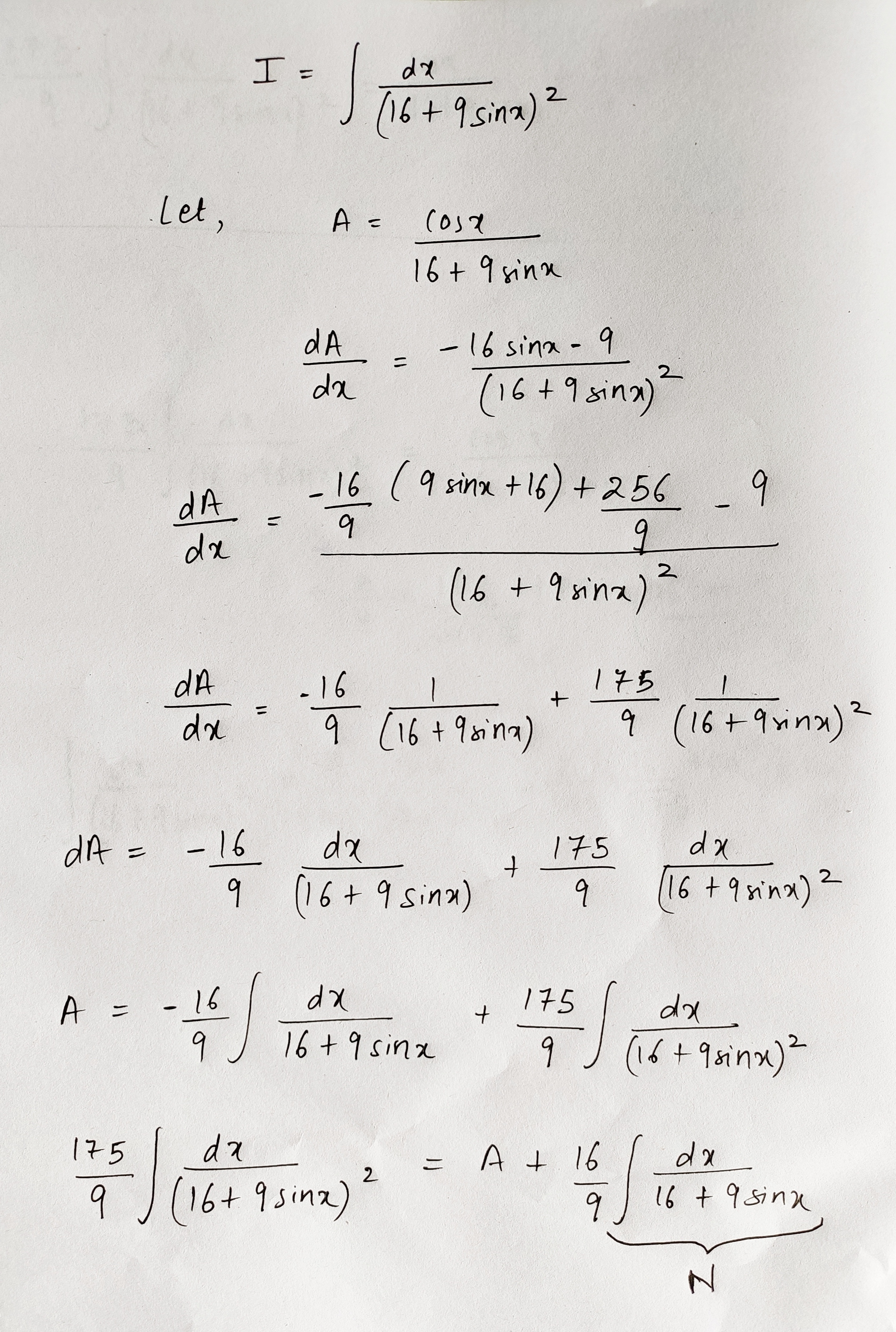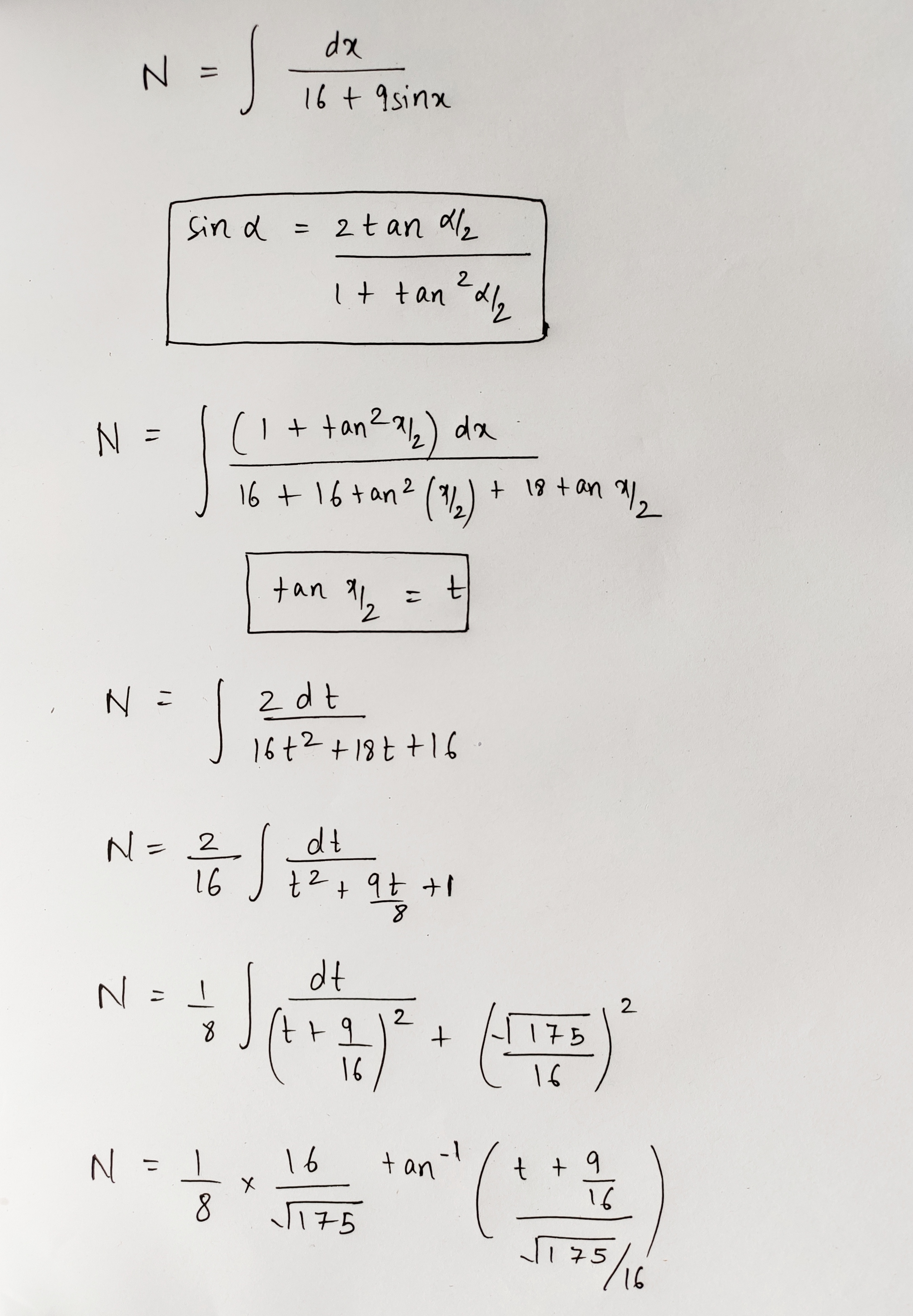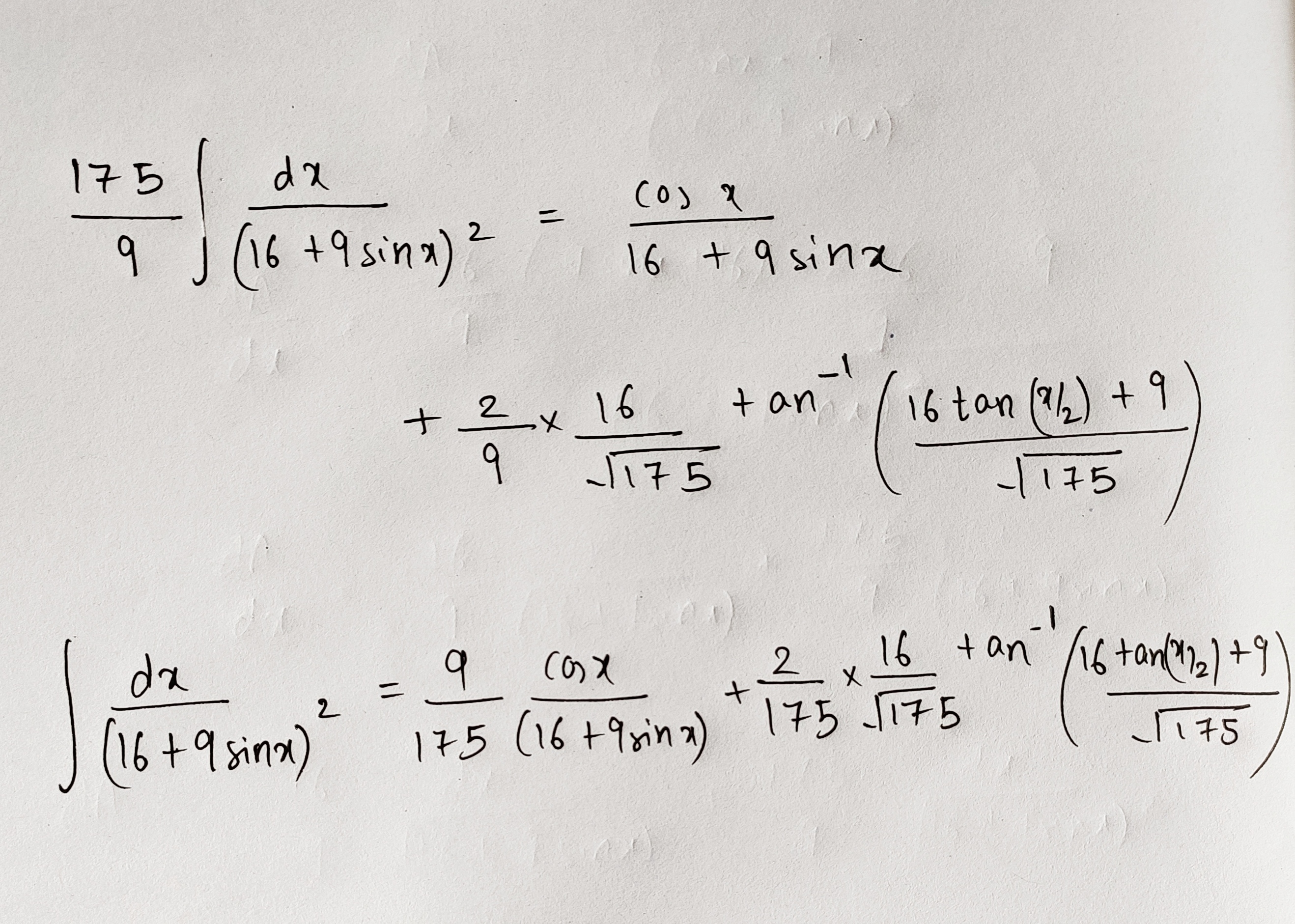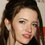# Luscious Integration

Here is a problem $I=\int \frac{1}{(16+9 \sin x) ^{2}}dx$
Here is the solution$\large +C$

Feel fee to ask anything regarding my solution.Note by Talulah Riley
10 months, 1 week ago

This discussion board is a place to discuss our Daily Challenges and the math and science related to those challenges. Explanations are more than just a solution — they should explain the steps and thinking strategies that you used to obtain the solution. Comments should further the discussion of math and science.

When posting on Brilliant:

• Use the emojis to react to an explanation, whether you're congratulating a job well done , or just really confused .
• Ask specific questions about the challenge or the steps in somebody's explanation. Well-posed questions can add a lot to the discussion, but posting "I don't understand!" doesn't help anyone.
• Try to contribute something new to the discussion, whether it is an extension, generalization or other idea related to the challenge.

MarkdownAppears as
*italics* or _italics_ italics
**bold** or __bold__ bold
- bulleted- list
• bulleted
• list
1. numbered2. list
1. numbered
2. list
Note: you must add a full line of space before and after lists for them to show up correctly
paragraph 1paragraph 2

paragraph 1

paragraph 2

[example link](https://brilliant.org)example link
> This is a quote
This is a quote
    # I indented these lines
# 4 spaces, and now they show
# up as a code block.

print "hello world"
# I indented these lines
# 4 spaces, and now they show
# up as a code block.

print "hello world"
MathAppears as
Remember to wrap math in $$ ... $$ or $ ... $ to ensure proper formatting.
2 \times 3 $2 \times 3$
2^{34} $2^{34}$
a_{i-1} $a_{i-1}$
\frac{2}{3} $\frac{2}{3}$
\sqrt{2} $\sqrt{2}$
\sum_{i=1}^3 $\sum_{i=1}^3$
\sin \theta $\sin \theta$
\boxed{123} $\boxed{123}$

Sort by:

Here's an alternative approach.

Let $y = \frac\pi2 - x$. Then, the integral becomes $\displaystyle I = -\int \dfrac{dy}{(16 + 9\cos y)^2}$.

Using half angle tangent substitution, we have $t = \tan(\frac y2) , \cos y = \dfrac{1-t^2}{1+t^2}$ and $dy = \dfrac{2 \cdot dt}{1 + t^2}$. The integral transforms to

$\begin{array} { r c l} I &=& \displaystyle -2 \int\dfrac1{\left (16 + 9 \left(\frac{1-t^2}{1+t^2} \right)\right)^2} \cdot \dfrac1{1+t^2} \, dt \\\phantom 0 \\ &=& \displaystyle -2 \int \dfrac{1+t^2}{ (16(1+t^2) + 9(1-t^2) )^2} \, dt \\ \phantom 0 \\ &=& \displaystyle -2 \int \dfrac{t^2 + 1}{ (7t^2 + 25 )^2} \, dt \\ \phantom0 \\ &=& \displaystyle -\frac27 \int \dfrac{7t^2 + 7}{ (7t^2 + 25 )^2} \, dt \\ \phantom0 \\ &=& \displaystyle -\frac27 \int \dfrac{7t^2 + 25 - 18}{ (7t^2 + 25 )^2} \, dt \\ \phantom0 \\ &=& \displaystyle -\frac27 \int \dfrac1{ 7t^2 + 25 } \, dt + \dfrac{36}{7} \int \dfrac1{(7t^2 + 25)^2} \, dt \\ \phantom0 \\ \end{array}$

For both integrals, apply the trigonometric substitution, $\sqrt7 t = 5 \tan u \Rightarrow \sqrt7 = 5\sec^2 u \cdot \dfrac{du}{dt}$.

$\begin{array} {r c l} I &=& \displaystyle -\frac27 \int \dfrac1{25\sec^2 u} \cdot \dfrac5{\sqrt7} \sec^2 u \, du + \dfrac{36}{7} \int \dfrac1{(25\sec^2 u)^2} \cdot \dfrac5{\sqrt7} \sec^2 u \, du \\ \phantom 0 \\ &=& \displaystyle -\frac2{35\sqrt7} \int du + \dfrac{36}{875\sqrt7} \int \cos^2 u \, du \\ \phantom 0 \\ &=& \displaystyle -\frac2{35\sqrt7} u + \dfrac{36}{875\sqrt7} \cdot \frac14 (\sin(2u) + 2u) +C \\ \phantom 0 \\ &=& \displaystyle -\frac{32}{875\sqrt7} u + \dfrac{9}{875\sqrt7} \sin(2u) + C \\ \phantom 0 \\ \end{array}$

What's left is to back-substitute. $u = \tan^{-1} \left( \frac{\sqrt7}5 t\right)= \tan^{-1} \left( \frac{\sqrt7}5 \tan\left( \frac y2\right)\right)= \tan^{-1} \left( \frac{\sqrt7}5 \tan\left( \frac {\pi /2 - x}2\right)\right)$. Finishing it off with $\tan\left( \frac {\pi /2 - x}2\right) = \dfrac{1 - \tan(\frac x2)}{1 + \tan(\frac x2)}$, we get:

$I = \displaystyle -\frac{32}{875\sqrt7} \tan^{-1} \left( \frac{\sqrt7}5 \tan\left( \dfrac{1 - \tan(\frac x2)}{1 + \tan(\frac x2)} \right)\right) + \dfrac{9}{875\sqrt7} \sin \left [ 2\left( \tan^{-1} \left( \frac{\sqrt7}5 \tan\left( \dfrac{1 - \tan(\frac x2)}{1 + \tan(\frac x2)} \right)\right) \right) \right] + C$

- 10 months, 1 week ago

@Pi Han Goh very very nice. Thanks for sharing.
By the way, do you know I am Neeraj . Due to some reason I have changed my name

- 10 months, 1 week ago

Now I know.

- 10 months, 1 week ago

@Pi Han Goh your integration skills are very nice.

- 10 months, 1 week ago

Nice one. I like it.

- 10 months, 1 week ago

@Pi Han Goh Btw have you ever participated in an Integration bee or an Integration competition? Holy shit... you're really good!

- 10 months, 1 week ago

No, but I'm aware of these competitions.

- 10 months, 1 week ago

@Pi Han Goh how to solve this integration, is there any alternative methods also? , $\int_{π}^{2π} \frac{(x^{2}+2) \cos x}{x^{3}} dx$

- 10 months, 1 week ago

Try posting each distinct question in a different note. Otherwise, it gets really cluttered and messy to see what note is.

- 10 months, 1 week ago

@Pi Han Goh ok, I will post a note after one hour.

- 10 months, 1 week ago

@Pi Han Goh I have upvoted your solution here also, see above.

- 10 months ago

@Krishna Karthik Here it is, I'm going to bed now.

- 10 months, 1 week ago

@Steven Chase @Karan Chatrath , in case you guys are interested.

- 10 months, 1 week ago

Yeah; it's really impressive. Good job mate :)

- 10 months, 1 week ago

Here's another method:

The integrand can be expressed as $\dfrac{1 + 9\cos x - 9\cos x}{(16 + 9\sin x)^2} = \dfrac{1 + 9\cos x}{(16 + 9\sin x)^2} - \dfrac{9\sin x}{(16 + 9\sin x)^2}.$

Split this into two integrals, for the first integral, let $y = \frac \pi2 - x$ then $t = \tan(\frac y2)$, and you can solve it like how I've written in my other comment. And use $u = 16 + 9\sin x$ to solve the second integral.

- 10 months, 1 week ago

@Pi Han Goh Thank you so much

- 10 months, 1 week ago

Bruh that's fuggin awesome!!!!

- 10 months, 1 week ago

@Krishna Karthik Do you know above line?

- 10 months, 1 week ago

Sorry? Yeah; you're going to bed. Cya.

- 10 months, 1 week ago

@Krishna Karthik it is the style of Steven Sir.

- 10 months, 1 week ago

Lol I just love how you added a funny little "plus C" at the end lmao

- 10 months, 1 week ago

@Krishna Karthik i forgot to write it in page. But this seems me more beautiful.
Conclusion:Everything happens for good

- 10 months, 1 week ago

@Pi Han Goh @Krishna Karthik can you please help me in this integration $\int_{π}^{2π} \frac{(x^{2}+2) \cos x}{x^{3}} dx$

- 10 months, 1 week ago

@Lil Doug This one's actually quite easy. I'll post a solution soon.

- 10 months, 1 week ago

@Krishna Karthik please post it now.

- 10 months, 1 week ago

So, the integral can be rewritten as:

$\displaystyle \int \left( \frac{\cos(x)}{x} + \frac{2 \cos(x)}{x^3} \right) dx$

So, we can split it into two integrals, which we can do using integration by parts.

$\displaystyle \int \frac{\cos(x)}{x} dx + 2 \int \frac{\cos(x)}{x^3} dx$

So, we do integration by parts with the first half:

$\displaystyle u = \frac{1}{x}$, $\displaystyle dv = \cos(x)$

$\displaystyle du = - \frac{1}{x^2}$, $\displaystyle v = \sin(x)$

So, it can be rewritten as:

$\displaystyle I_1 = \frac{\sin(x)}{x} - \int \frac{\sin(x)}{x^2}$

Doing integration by parts for the second half, we get:

$\displaystyle I_2 = - \frac{\cos(x)}{2x^2} - \int \frac{\sin(x)}{x^2}$

Now simplifying, $\displaystyle \int \frac{\sin(x)}{x^2}$ cancels out, leaving:

$\displaystyle \frac{x \sin(x) - \cos(x)}{x^2} + C$

So substituting, you get:

$\displaystyle \boxed{ \frac{-5}{4 \pi^2}}$

- 10 months, 1 week ago

@Krishna Karthik how does it get cancel, both are negative, both will add up.

- 10 months, 1 week ago

Oh wait... shit. I fucked up. So, let's try to calculate that integral $\displaystyle \int \frac{\sin(x)}{x^2}$

- 10 months, 1 week ago

@Krishna Karthik what answer you are getting?

- 10 months, 1 week ago

I've posted the solution. It's just integration by parts.

- 10 months, 1 week ago

I suspect the indefinite integral is in the form of $A \cdot \dfrac{\sin x}x + B \cdot \dfrac{\sin x}{x^2} + C \cdot \dfrac{\cos x}{x} + D \cdot \dfrac{\cos x}{x^2},$ where $A,B,C,D$ are constants. Differentiating this expression with respect to $x$ should gives us back the integrand $\dfrac{(x^2 + 2)\cos x}{x^3}$.

Using quotient rule and comparing coefficients, we will get $(A,B,C,D) = (1,0,0,-1)$, thus $\int_\pi^{2\pi} \dfrac{ (x^2+2)\cos x}{x^3} \, dx = \left [ \dfrac{\sin x}x - \dfrac{\cos x}{x^2} \right ]_{\pi}^{2\pi} = \boxed{-\dfrac{5}{4\pi^2} } \approx-0.126651 .$

- 10 months, 1 week ago

@Krishna Karthik i am getting $I_{1} =\frac{\sin x}{x}+\int \frac{\sin x }{x^{2}} dx$

- 10 months, 1 week ago

You missed out the $\displaystyle -\frac{\cos(x)}{x^2}$ term.

- 10 months, 1 week ago

@Krishna Karthik where bro, I am. Telling $I_{1}$

- 10 months, 1 week ago

Oh oops; my bad. Yup. I meant the exact same thing as you. So they should cancel!

- 10 months, 1 week ago

Wait... how are you getting a positive $\displaystyle \int \frac{\sin(x)}{x^2}$? It should be negative because $\displaystyle -\frac{1}{x^2}$

- 10 months, 1 week ago

Btw, numerically integrating, I'm getting -0.12779

- 10 months, 1 week ago

@Krishna Karthik share the code also.

- 10 months, 1 week ago

I'm just using a standard midpoint rule calculator through python.

- 10 months, 1 week ago

@Krishna Karthik share the code means share the code.

- 10 months, 1 week ago

Lmao, ok. Coming up.

- 10 months, 1 week ago

@Lil Doug The code.

  1 2 3 4 5 6 7 8 9 10 11 12 13 14 15 16 17 18 19 20 21 22 23 24 25 26 27 28 29 30 31 32 33 34 35 36 37 import math def function(x): return ((x**2+2)*math.cos(x))/x**3 n=2500 b=6.28 a=3.14 deltaX=(b-a)/n def mean(a,b): meanValue=(a+b)/2 return meanValue xValue=[a] heightValue=[] for i in range(0,n): xValue.append(a+deltaX) deltaX=deltaX+(b-a)/n for i in xValue: heightValue.append(function(mean(i,i+(b-a)/n))) del heightValue[-1] the_sum=sum(heightValue) definite_integral=the_sum*((b-a)/n) print('Definite integral evaluated by midpoint rule: '+str(definite_integral)) 

- 10 months, 1 week ago

 1 2 3 4 5 6 7 from math import cos, pi def function(x): return ((x**2+2)* cos(x))/x**3 n = 2500 ; a = pi ; b = 2 * pi # The rest is the same. 

- 10 months, 1 week ago

@Pi Han Goh I don't like to code in single-line style. I prefer readability as a programmer, personally.

Usually, I prefer to indent functions so I can see what is inside the function clearer. And btw thx for the suggestion of using it as just cos and pi rather than specifying the library.

- 10 months ago

@Krishna Karthik No matter whatever you like, First upvote his alternative method of this note integration above.

- 10 months ago

@Lil Doug Oh yeah; sorry I forgot to. You can't upvote subcomments, though, sadly.

- 10 months ago

@Krishna Karthik They are not cancelling bro
I think you have copied the solution and just paste it here.
Not taken a look on the solution

- 10 months, 1 week ago

No, I looked. And you're right; they're not cancelling. My integration by parts has failed. We should work out $\displaystyle \int \frac{\sin(x)}{x^2}$

- 10 months, 1 week ago

@Krishna Karthik it is not your integration by parts .
It is the mistake of this website https://www.integral-calculator.com/ who told you wrong method

- 10 months, 1 week ago

Integral calculator? I worked most of this out by hand; I tried integrating by parts. I checked with integral calculator because I got stuck midway; it mistakingly said it would cancel out (integral calculator can be stupid sometimes...) so I copied an integration by parts method from them. Maybe there's some weird trig substitution or something that I'm missing. I'll try the problem soon.

- 10 months, 1 week ago

@Krishna Karthik. you code is also not working

- 10 months, 1 week ago

It is. Show me your screen so I can debug for you.

- 10 months, 1 week ago

Btw the my midpoint rule calculator seems to be coming up with a reasonable answer. This is actually a tricky problem... maybe integration by parts isn't the trick. Ask Pi Han Goh.

- 10 months, 1 week ago

@Lil Doug I just posted a new note; it's a photo of the working code.

- 10 months, 1 week ago

@Pi Han Goh here above you can see

- 9 months, 3 weeks ago

A few pointers:

• Why start with $A = \cdots$ (yes, I know the answer to this question). But you seem to pull it out of thin air without any justification.

• Say you used quotient rule, otherwise, the newbies can't follow this reasoning.

• At least motivate why you want to work on the differentiation of $A$ first, by first explaining that the original integrand needs to be broken down (by considering a lesser power in the denominator).

• Where did your $\sin \alpha =\cdots$ come from ? (I know the answer to this question too) It seems mystifying to the uninitiated.

• At least make it explicit why you want convert the integrand in the form of $\frac1{t^2 + a^2}$ in the final step.

• Handwriting issue: Some of your brackets does not properly encapsulate the required expressions.

• Handwriting issue: Some of your fractions' horizontal line should be longer.

- 9 months, 3 weeks ago